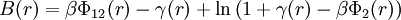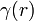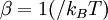# Soft-core mean spherical approximation

Jump to: navigation, search

Junzo Chihara (along with Madden and Rice) extended the Percus Yevick equation for Lennard-Jones systems to provide a soft-core mean spherical approximation, the SMSA,$B(r) = \beta \Phi_{12} (r) - \gamma (r) + \ln \left( 1 + \gamma (r) - \beta \Phi_2 (r) \right)$

where$\Phi_{12} (r)$ is the pair potential,$\gamma (r)$ is the indirect correlation function, and$\beta = 1(/k_B T)$, where$k_B$ is the Boltzmann constant.